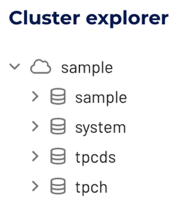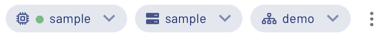# Learn SQL decision logic #

Welcome to the Learn SQL decision logic tutorial, where you explore useful ways to craft your SQL statements using the Sample dataset. Using Conditional expressions, implement decision logic within each SQL statement.

## Requirements #

To complete the tutorial using Starburst Galaxy, you must create a free trial or activate your user account.

Once you login to Starburst Galaxy, the `sample` cluster and `sample` catalog are usually pre-configured and ready for querying.

• If you do not see the `sample` catalog automatically available, create a Sample dataset catalog with the name `sample`.
• If you do not see the `sample` cluster automatically available, create a cluster named `sample` and add the `sample` catalog.

In the query editor, navigate to the Cluster explorer.

1. In the navigation menu, expand the `sample` cluster to view its catalogs.
2. Select the `sample` catalog.In the location drop-downs, select the cluster `sample` and the catalog `sample` in order to run the queries without having to specify the full table path location in each query.The namespace for a table is typically specified as `catalog_name.schema_name.table_name`. For the predefined sample dataset, this configuration is as follows:

``````sample.demo.<table_name>
``````

If you choose to name your catalog differently, either adjust the queries in the tutorials accordingly or select the appropriate catalog in the location drop-downs.

If you choose to name your cluster differently, select the appropriate cluster in the location drop-downs.

## Conditional expressions #

Conditional expressions are used to define logic based on an appropriately satisfied condition. Before implementing the Conditional expressions, count the number of missions associated with each astronaut.

``````SELECT
name,
count() AS nbr_missions
FROM
sample.demo.astronauts
GROUP BY
name
ORDER BY
nbr_missions DESC;
``````

Classify the astronauts as either rookies or veterans using the `IF` expression and the `count()` aggregate function.

``````SELECT
name,
count() AS nbr_of_missions,
IF(count() > 1, 'Veteran', 'Rookie') AS nbr_of_mission_qualification
FROM
sample.demo.astronauts
GROUP BY
name;
``````

Expand on the astronaut mission classification by assigning a space rank based on the specific number of completed of missions. Use the `CASE` expression to assign the new astronaut mission classification. Since there are multiple entries for each astronaut to correspond to the number of completed missions, observe the astronauts rise through the space ranks for each completed mission.

``````SELECT
name,
nationality,
mission_number,
CASE
WHEN mission_number < 3 THEN 'Space Cadet'
WHEN mission_number = 3 THEN 'Space Captain'
WHEN mission_number = 4 THEN 'Space Colonel'
WHEN mission_number = 5 THEN 'Space General'
WHEN mission_number = 6 THEN 'Space Warrior'
WHEN mission_number > 6 THEN 'Space Avenger'
ELSE 'unknown'
END AS space_rank
FROM
sample.demo.astronauts
ORDER BY
name,
mission_number;
``````

Observe the trajectory of one of the space avengers as he rose through the space ranks for each completed mission.

``````SELECT
name,
nationality,
mission_number,
year_of_mission,
mission_title,
CASE
WHEN mission_number < 3 THEN 'Space Cadet'
WHEN mission_number = 3 THEN 'Space Captain'
WHEN mission_number = 4 THEN 'Space Colonel'
WHEN mission_number = 5 THEN 'Space General'
WHEN mission_number = 6 THEN 'Space Warrior'
WHEN mission_number > 6 THEN 'Space Avenger'
ELSE 'unknown'
END AS space_rank
FROM
sample.demo.astronauts
WHERE
name = 'Ross, Jerry L.'
ORDER BY
name,
mission_number;
``````

Instead of assigning a space rank for multiple entries of the same astronaut, use the `GROUP BY` clause to calculate the space rank of each astronaut from their completed total number of missions.

``````SELECT
name,
total_number_of_missions,
CASE
WHEN total_number_of_missions < 3 THEN 'Space Cadet'
WHEN total_number_of_missions = 3 THEN 'Space Captain'
WHEN total_number_of_missions = 4 THEN 'Space Colonel'
WHEN total_number_of_missions = 5 THEN 'Space General'
WHEN total_number_of_missions = 6 THEN 'Space Warrior'
WHEN total_number_of_missions > 6 THEN 'Space Avenger'
ELSE 'unknown'
END AS space_rank
FROM
sample.demo.astronauts
GROUP BY
name, total_number_of_missions
ORDER BY
total_number_of_missions DESC;
``````

Only view astronauts of the rank of ‘Space Colonel’ or higher by adding the `WHERE` clause to the query.

``````SELECT
name,
total_number_of_missions,
CASE
WHEN total_number_of_missions < 3 THEN 'Space Cadet'
WHEN total_number_of_missions = 3 THEN 'Space Captain'
WHEN total_number_of_missions = 4 THEN 'Space Colonel'
WHEN total_number_of_missions = 5 THEN 'Space General'
WHEN total_number_of_missions = 6 THEN 'Space Warrior'
WHEN total_number_of_missions > 6 THEN 'Space Avenger'
ELSE 'unknown'
END AS space_rank
FROM
sample.demo.astronauts
WHERE
total_number_of_missions >= 4
GROUP BY
name, total_number_of_missions
ORDER BY
total_number_of_missions DESC;
``````

## Next steps #

Check out our other tutorials, or dive right into the SQL documentation and experiment with your own data.